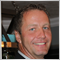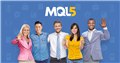# Problem with CreateObject122

I created an indicator that measures the IBS (internal bar strength), I used part of the function listed in MQL docs but I do have a problem when I switch time frames, it doesn't seem to run the OnDeinit to clear up already created objects before it starts creating new objects based on the new timeframe, which I thought it was supposed to do. The result is that I get Error Code 4200 because the object name already exist because it wasn't removed correctly. I'm going blind trying to figure out what I'm missing but cannot figure it out (yet). Enclosed is the code, I would appreciate any assistance in resolving it.

```//+------------------------------------------------------------------+

//|                                                          IBS.mq4 |

//|                        Copyright 2021, MetaQuotes Software Corp. |

//|                                             https://www.mql5.com |

//+------------------------------------------------------------------+

#property version   "1.10"

#property description "Internal Bar Strength (IBS) Indicator. Buy when IBS is less than 0.20 and sell when its above 0.70"

#property strict

#property indicator_chart_window

// Defining arrays to use with indicator

double ibs[];

double myPoint;

//--- input parameters of the indicator

input double            InpSell=0.70;            // What level to use for Sell, will be colored RED

input double            InpBuy=0.20;             // What level to use for Buy, will be colored GREEN

input string            InpFont="Arial";         // Font

input int               InpFontSize=10;          // Font size

input color             InpColor=clrRed;         // Color

input double            InpAngle=90.0;           // Slope angle in degrees

input ENUM_ANCHOR_POINT InpAnchor=ANCHOR_LEFT;   // Anchor type

input bool              InpBack=false;           // Background object

input bool              InpSelection=false;      // Highlight to move

input bool              InpHidden=true;          // Hidden in the object list

input long              InpZOrder=0;             // Priority for mouse click

//+------------------------------------------------------------------+

//| Custom indicator initialization function                         |

//+------------------------------------------------------------------+

int OnInit()

{

//---

int obj_total=ObjectsTotal();

PrintFormat("Total %d objects",obj_total);

for(int i=obj_total-1; i>=0; i--)

{

string name=ObjectName(i);

PrintFormat("Deleteing object %d: %s",i,name);

ObjectDelete(name);

}

myPoint = Point();

if(Digits() == 5 || Digits() == 3)

{

myPoint *= 10;

}

//---

return(INIT_SUCCEEDED);

}

//+------------------------------------------------------------------+

//|                                                                  |

//+------------------------------------------------------------------+

void OnDeinit(const int reason)

{

//---

int obj_total=ObjectsTotal();

PrintFormat("Total %d objects",obj_total);

for(int i=obj_total-1; i>=0; i--)

{

string name=ObjectName(i);

PrintFormat("Removing object %d: %s",i,name);

ObjectDelete(name);

}

//---

}

//+------------------------------------------------------------------+

//|                                                                  |

//+------------------------------------------------------------------+

bool TextCreate(const long chart_ID = 0,      // chart's ID

const string name="IBS",     // object name

const int sub_window=0,      // subwindow index

datetime   time=0,           // anchor point time

double price = 0,            // anchor point price

const string text="IBS",     // the text itself

const string font="Arial",

const int font_size=10,

color clr=clrRed,

const double angle=0.0,

const ENUM_ANCHOR_POINT anchor=ANCHOR_LEFT_UPPER,

const bool back=false,

const bool selection=false,

const bool hidden=true,

const long z_order=0)

{

//-- set anchor point coordinates if they are not set

ChangeTextEmptyPoint(time,price);

//-- reset the error value

ResetLastError();

//-- create Text object

if(!ObjectCreate(chart_ID,name, OBJ_TEXT,sub_window,time,price))

{

Print(__FUNCTION__,

": Failed to create ",name," object! Error code = ",GetLastError());

return(false);

}

//-- set the text

ObjectSetString(chart_ID,name,OBJPROP_TEXT,text);

//-- set text font

ObjectSetString(chart_ID,name,OBJPROP_FONT,font);

//-- set font size

ObjectSetInteger(chart_ID,name,OBJPROP_FONTSIZE, font_size);

//-- set the slope angle of the text

ObjectSetDouble(chart_ID,name,OBJPROP_ANGLE,angle);

//-- set anchor type

ObjectSetInteger(chart_ID,name,OBJPROP_ANCHOR,anchor);

//-- set color

ObjectSetInteger(chart_ID,name,OBJPROP_COLOR, clr);

//-- dislay in the foreground (false) or background (true)

ObjectSetInteger(chart_ID,name,OBJPROP_BACK, back);

//-- enable (true) or disable (false) the mode of moving the object by mouse

ObjectSetInteger(chart_ID,name,OBJPROP_SELECTABLE, selection);

ObjectSetInteger(chart_ID,name,OBJPROP_SELECTED, selection);

//-- hide (true) or display (false) graphical object name in the object list

ObjectSetInteger(chart_ID,name,OBJPROP_HIDDEN,hidden);

//-- set the priority for receiving the event of a mouse click in the chart

ObjectSetInteger(chart_ID,name,OBJPROP_ZORDER,z_order);

return(true);

}

//+------------------------------------------------------------------+

//| Check anchor point values and set default values                 |

//| for empty ones                                                   |

//+------------------------------------------------------------------+

void ChangeTextEmptyPoint(datetime &time,double &price)

{

//--- if the point's time is not set, it will be on the current bar

if(!time)

time=TimeCurrent();

//--- if the point's price is not set, it will have Bid value

if(!price)

price=SymbolInfoDouble(Symbol(),SYMBOL_BID);

}

//+------------------------------------------------------------------+

//| Custom indicator iteration function                              |

//+------------------------------------------------------------------+

int OnCalculate(const int rates_total,

const int prev_calculated,

const datetime &time[],

const double &open[],

const double &high[],

const double &low[],

const double &close[],

const long &tick_volume[],

const long &volume[],

{

//--- Reset the error value

ResetLastError();

double IBS = 0;

color ibsColor = InpColor;

string ibsFont = InpFont;

double ibsAngle = InpAngle;

int total = 0;

if(prev_calculated==0)

{

total=rates_total-2;

}

else

{

total = rates_total - prev_calculated;

}

for(int i=0; i<total; i++)

{

//         tops[i] = High[i];

//         bottoms[i] = Low[i];

//

double range1 = 0;

double range2 = 0;

IBS = 0;

if(High[i+1] < High[i+2] && Low[i+1] > Low[i+2])

{

range1 = Close[i+1] - Low[i+1];

range2 = High[i+1] - Low[i+1];

IBS = range1 / range2;

{

ibsColor = clrGreen;

ibsFont = "Arial Bold";

ibsAngle = InpAngle;

}

else

if(IBS > InpSell)

{

ibsColor = clrRed;

ibsFont = "Arial Bold";

ibsAngle = InpAngle;

}

else

if(IBS > InpBuy && IBS < InpSell)

{

ibsColor = clrBlack;

ibsFont = "Arial";

ibsAngle = 90;

}

if(!TextCreate(0,"IBS_"+(string)i,0,time[i+1],high[i+1]+1 * myPoint,DoubleToStr(IBS,3),ibsFont,InpFontSize,ibsColor,ibsAngle,InpAnchor,InpBack,InpSelection,InpHidden,InpZOrder))

{

return(true);

}

int obj_total=ObjectsTotal();

PrintFormat("Total %d objects",obj_total);

for(int j=obj_total-1; j>=0; j--)

{

string name=ObjectName(j);

PrintFormat("IBS object %d: %s",j,name);

}

//if (range2 == 0)

//{

//   range2 = range2 + 0.01;

//}

//tops[i] = High[i];

//           double IBS = range1 - range2;

//           ibs[i] = High[i];

ChartRedraw();

}

}

return(rates_total);

}

//+------------------------------------------------------------------+

```Discover new MetaTrader 5 opportunities with MQL5 community and services
• www.mql5.com26425

Christer Wittusen: it doesn't seem to run the OnDeinit to clear up already created objects before it starts creating new objects based on the new timeframe, which I thought it was supposed to do. The result is that I get Error Code 4200 because the object name already exist because it wasn't removed correctly.
1. That is not the problem. You did delete them.

2. `if(!TextCreate(0,"IBS_"+(string)i,0`

You can't use an as-series index in object names as they are not unique. As soon as a new bar starts, you will be trying to create a new name (e.g. "name0"), same, existing, previous, name (e.g. “name0” now on bar one.)

Use time (as int) or a non-series index:

`#define  SERIES(I)   (Bars - 1 - I) // As-series to non-series or back.`
-122

William Roeder #:
1. That is not the problem. You did delete them.

2. You can't use an as-series index in object names as they are not unique. As soon as a new bar starts, you will be trying to create a new name (e.g. "name0"), same, existing, previous, name (e.g. “name0” now on bar one.)

Use time (as int) or a non-series index:

-

William:
Thank you, I do get object names as a series, like IBS_01, IBS_02, but I guess something prevents it from being deleted when switching TFs, however, it seems most of them are being deleted. However, I think you probably know more than I do, how would you replace my current code then because I'm not quite following how to fix the code with what you suggested.

Regards,

Chris26425

Christer Wittusen #: but I guess something prevents it from being deleted when switching TFs,

What part of “that is not the problem. You did delete them” was unclear?

Christer Wittusen #: how would you replace my current code then because I'm not quite following how to fix the code with what you suggested.
I gave you two suggestions. Modify your code.122

William Roeder #:

What part of “that is not the problem. You did delete them” was unclear?

I gave you two suggestions. Modify your code.

I re-read your thing and I understand that I'm successfully removing the objects, however, that candle 0 and 1 will always cause issues and now I'm trying to figure out how to do a non-series index or time, I'm still new at this code (mql) so I'm still learning. I'll figure it out, thx for your help.

/Chris